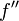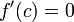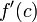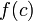# Difference between revisions of "Second derivative test"

## Statement

### What this test is for

This test is a partial test (i.e., it may be inconclusive) for determining whether a given critical point for a function is a point of local minimum, point of local maximum, or neither.

### What the test says

Suppose$f$ is a function and$c$ is a point in the interior of the domain of$f$, i.e.,$f$ is defined on some open interval containing$c$. Suppose, further, that$f''$, i.e., the second derivative of$f$, exists at$c$. Suppose also that$f'(c)=0$, so$c$ is a critical point for$f$. Then:

Hypothesis on$f'(c)$ Hypothesis on$f''(c)$ Conclusion$f'(c) =0$$f''(c) < 0$$f$ attains a local maximum value at$c$ (the value is$f(c)$)$f'(c) = 0$$f''(c) > 0$$f$ attains a local minimum value at$c$ (the value is$f(c)$)$f'(c) = 0$$f''(c) = 0$ The test is inconclusive.$f$ may attain a local maximum value, a local minimum value, have a point of inflection, or have some different behavior at the point$c$.

### Relation with critical points

The second derivative test is specifically used only to determine whether a critical point where the derivative is zero is a point of local maximum or local minimum. Note in particular that:

• For the other type of critical point, namely that where$f'$ is undefined, the second derivative test cannot be used.
• Since point of local extremum implies critical point, we don't have to worry about points that are not critical points -- none of them will give local extrema.

## Strength of the test

### Second derivative test requires twice differentiability at but not around the point

The second derivative test can be applied at a critical point$c$ for a function$f$ only if$f$ is twice differentiable at$c$. This in particular forces$f$ to be once differentiable around$c$.

However, the test does not require the second derivative$f''$ to be defined around$c$ or to be continuous at$c$.

### Relation with first derivative test

For further information, refer: second derivative test operates via first derivative test, second derivative test is not stronger than first derivative test

The first derivative test is strictly more powerful than the second derivative test, i.e., whenever the second derivative test is applicable and conclusive, so is the first derivative test. However, there can be situations where the first derivative test is conclusive but the second derivative test is not.

## Ease of use

The second derivative test is strictly less powerful than the first derivative test, so why is it ever used? The main reason is that in cases where it is conclusive, the second derivative test is often easier to apply. This, in turn, is because the second derivative test only requires the computation of formal expressions for derivatives and evaluation of the signs of these expressions at a point rather than on an interval. Evaluation at a point often requires less symbolic/algebraic manipulation.

## When is the test conclusive and inconclusive?

### The test can never be conclusive about the absence of local extrema

The first derivative test can sometimes conclusively establish that a given critical point is not a point of local extremum. The second derivative test can never conclusively establish this. It can only conclusively establish affirmative results about local extrema.

### Situations when the test is inconclusive

The second derivative test is inapplicable or inconclusive in the following situations. We denote the function by$f$ and the critical point by$c$:

What problem do we run into? What kind of trouble do we have? Can we use the first derivative test? Remedy that may work (other than reverting to the first derivative test)
The critical point is of the type where$f'$ is undefined We cannot take the second derivative, because even the first derivative doesn't exist. We may or may not be able to use the first derivative test. It is possible to construct examples of (local min + conclusive first derivative test), (local max + conclusive first derivative test), (neither + conclusive first derivative test), (local min + inconclusive first derivative test), (local max + inconclusive first derivative test), (neither + inconclusive first derivative test)$f'(c) = 0$ but$f''(c)$ is undefined We cannot take the second derivative at the critical point. We may or may not be able to use the first derivative test. It is possible to construct examples of (local min + conclusive first derivative test), (local max + conclusive first derivative test), (neither + conclusive first derivative test), (local min + inconclusive first derivative test), (local max + inconclusive first derivative test), (neither + inconclusive first derivative test) one-sided version of second derivative test: If the one-sided derivatives of$f'$ exist at$c$, then we can try checking that both one-sided derivatives of$f'$ have the stipulated sign for$f''$.$f'(c) = 0$ and$f''(c) = 0$ We are in the inconclusive case of the test as stated. We may or may not be able to use the first derivative test. It is possible to construct examples of (local min + conclusive first derivative test), (local max + conclusive first derivative test), (neither + conclusive first derivative test), (local min + inconclusive first derivative test), (local max + inconclusive first derivative test), (neither + inconclusive first derivative test) higher derivative tests